# ML Aggarwal Solutions for Class 8 Maths Chapter 16 - Symmetry Reflection and Rotation

ML Aggarwal Solutions for Class 8 Maths Chapter 16 – Symmetry Reflection and Rotation are provided here to help students prepare and grasp the concepts easily to score well in their board exams. This chapter mainly deals with problems based on lines of symmetry. Students who wish to have strong command over the subject can refer to ML Aggarwal Solutions. The problems here are formulated by our expert faculty team with utmost care to help students understand the concepts clearly. From the exam point of view, the solutions are designed in simple language which helps in scoring high marks in the board exams. The solutions PDF is available for students to easily download for free from the links given below and can start practising offline as well.

Chapter 16 – Symmetry Reflection and Rotation contains one exercise and objective type questions for students to practice the problems. The ML Aggarwal Class 8 Solutions present in this page provides solutions to questions related to each topic present in this chapter.

## Download the Pdf of ML Aggarwal Solutions for Class 8 Maths Chapter 16 – Symmetry Reflection and Rotation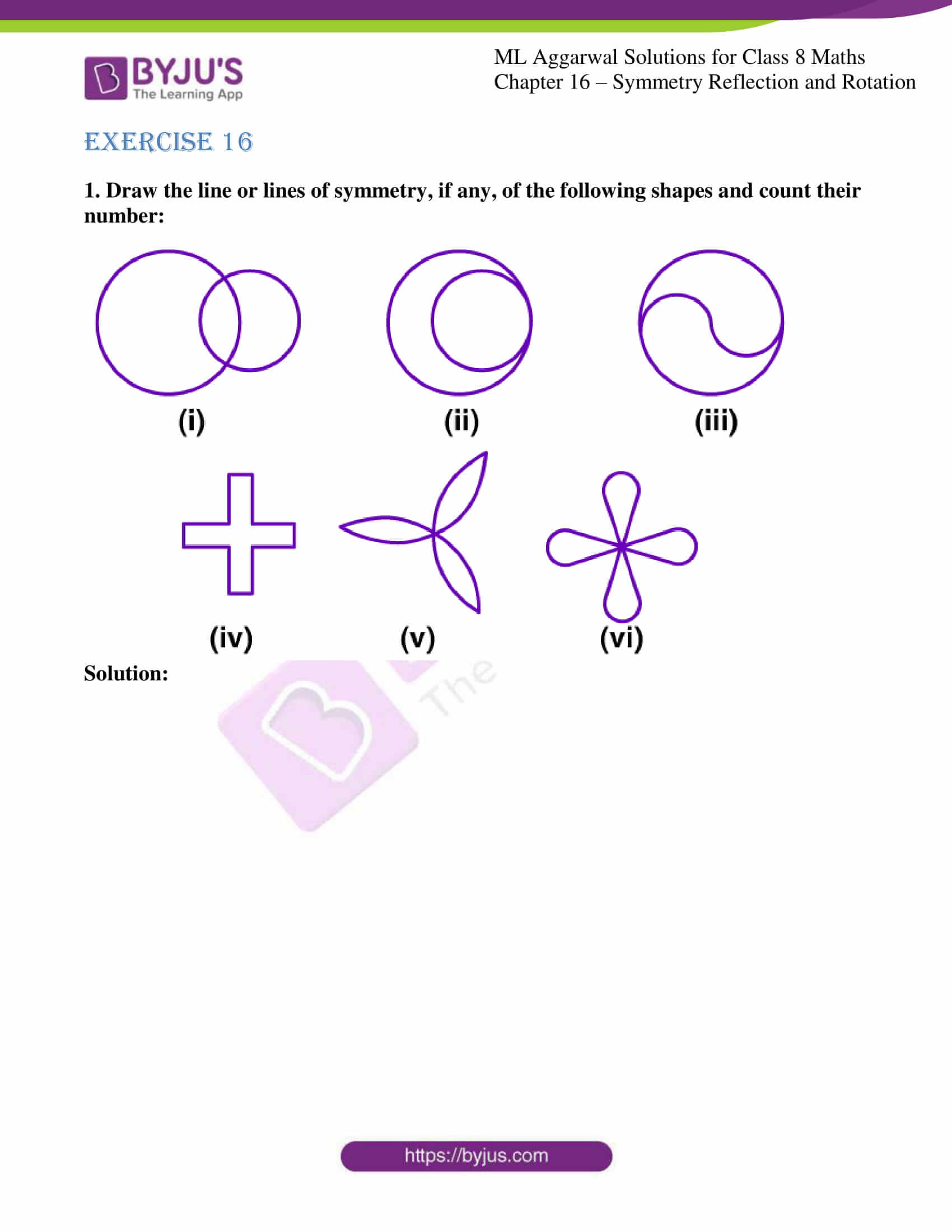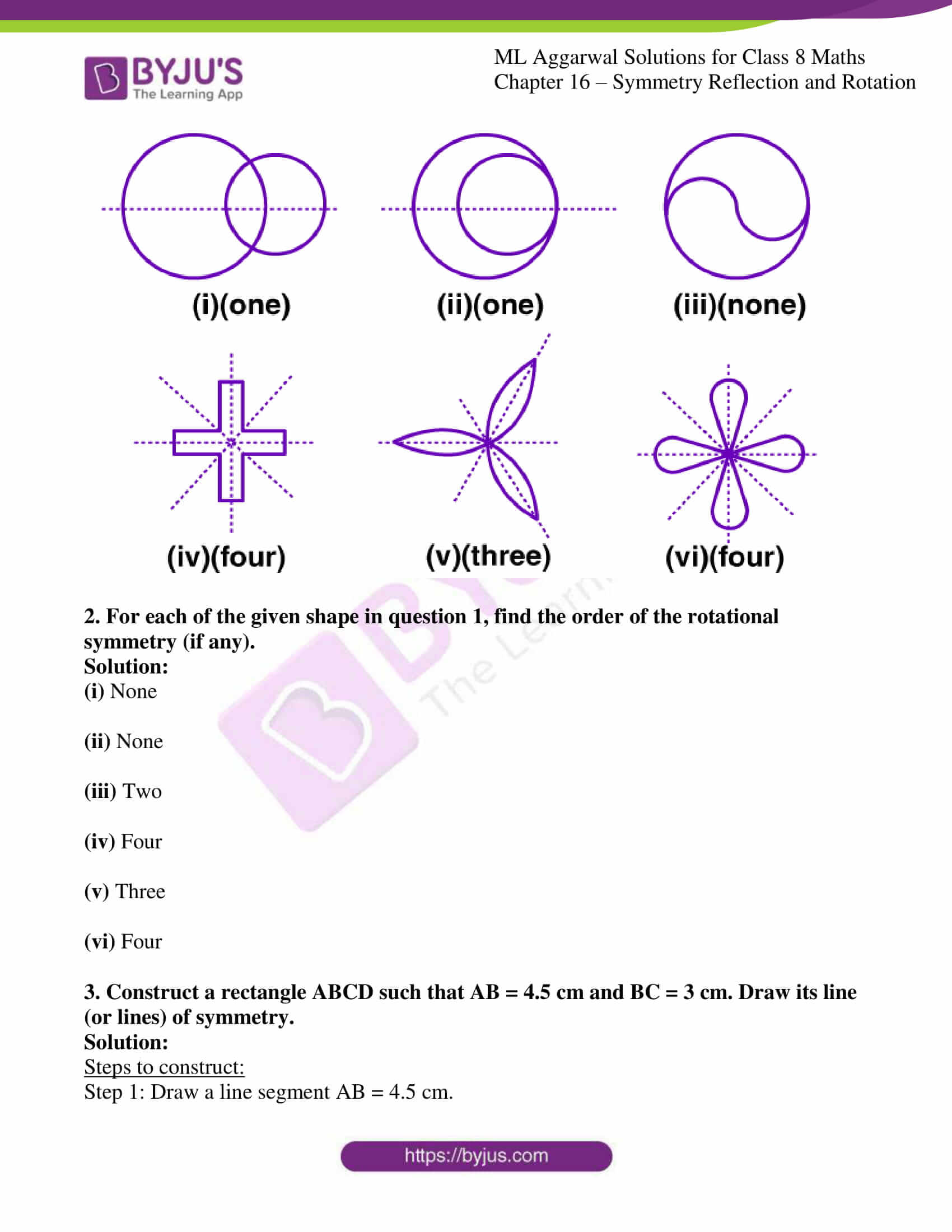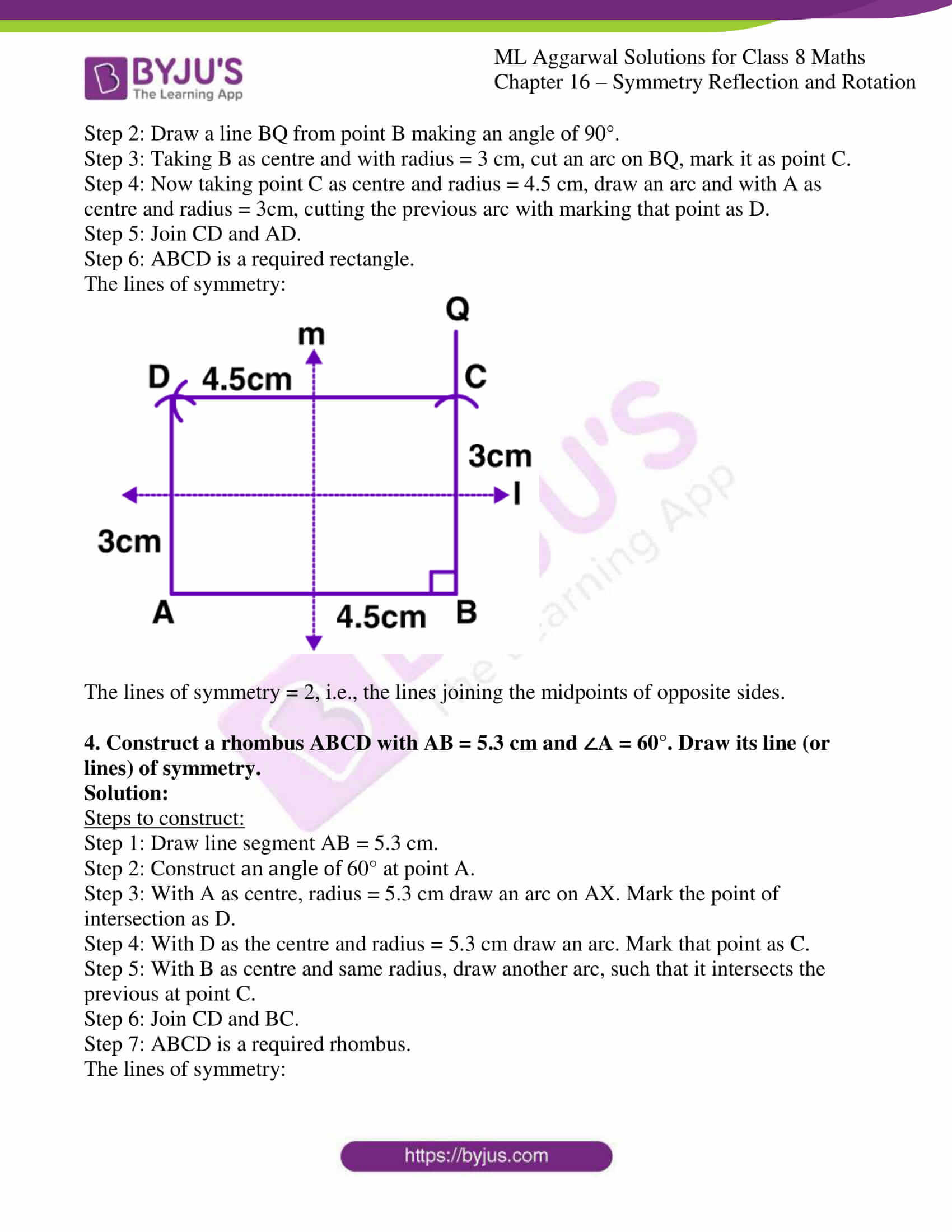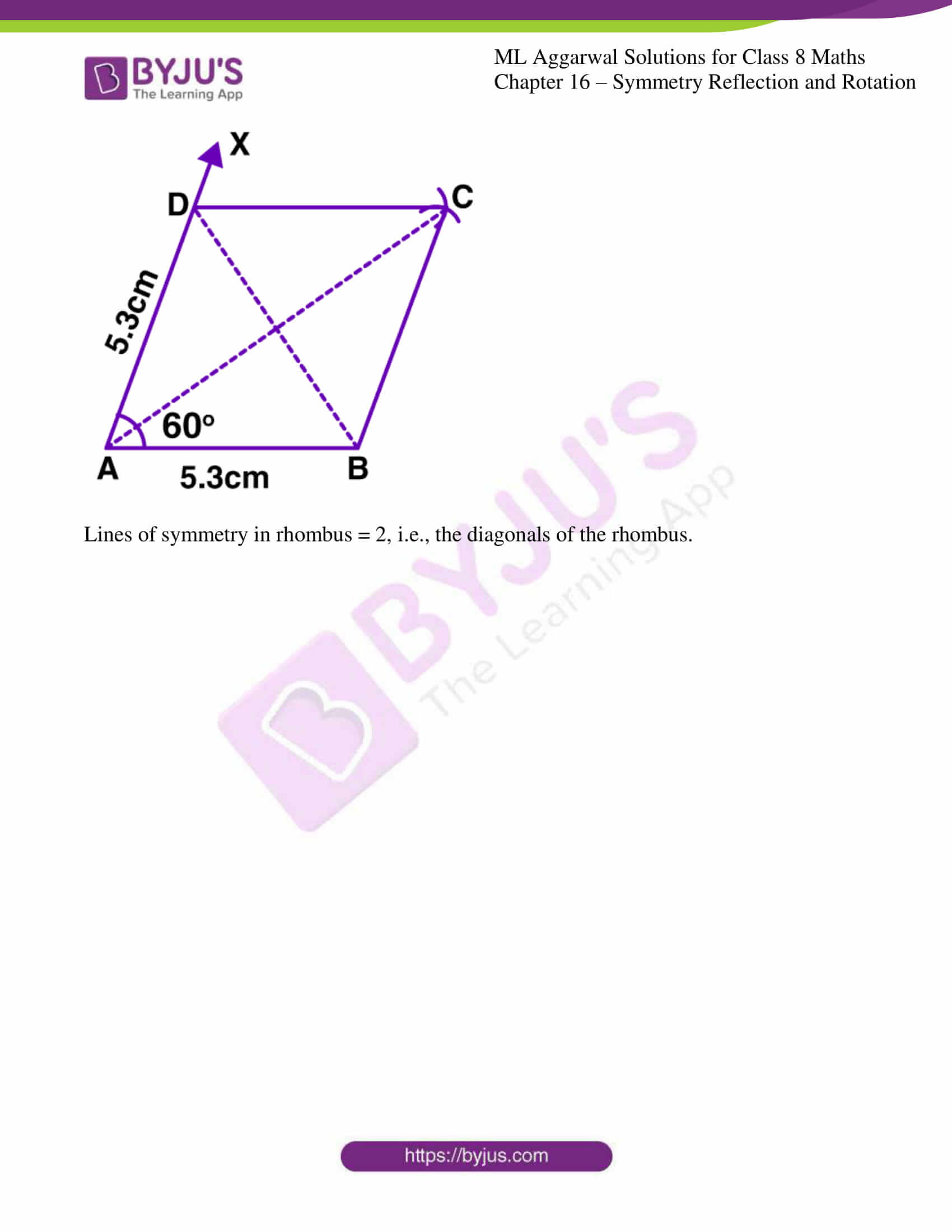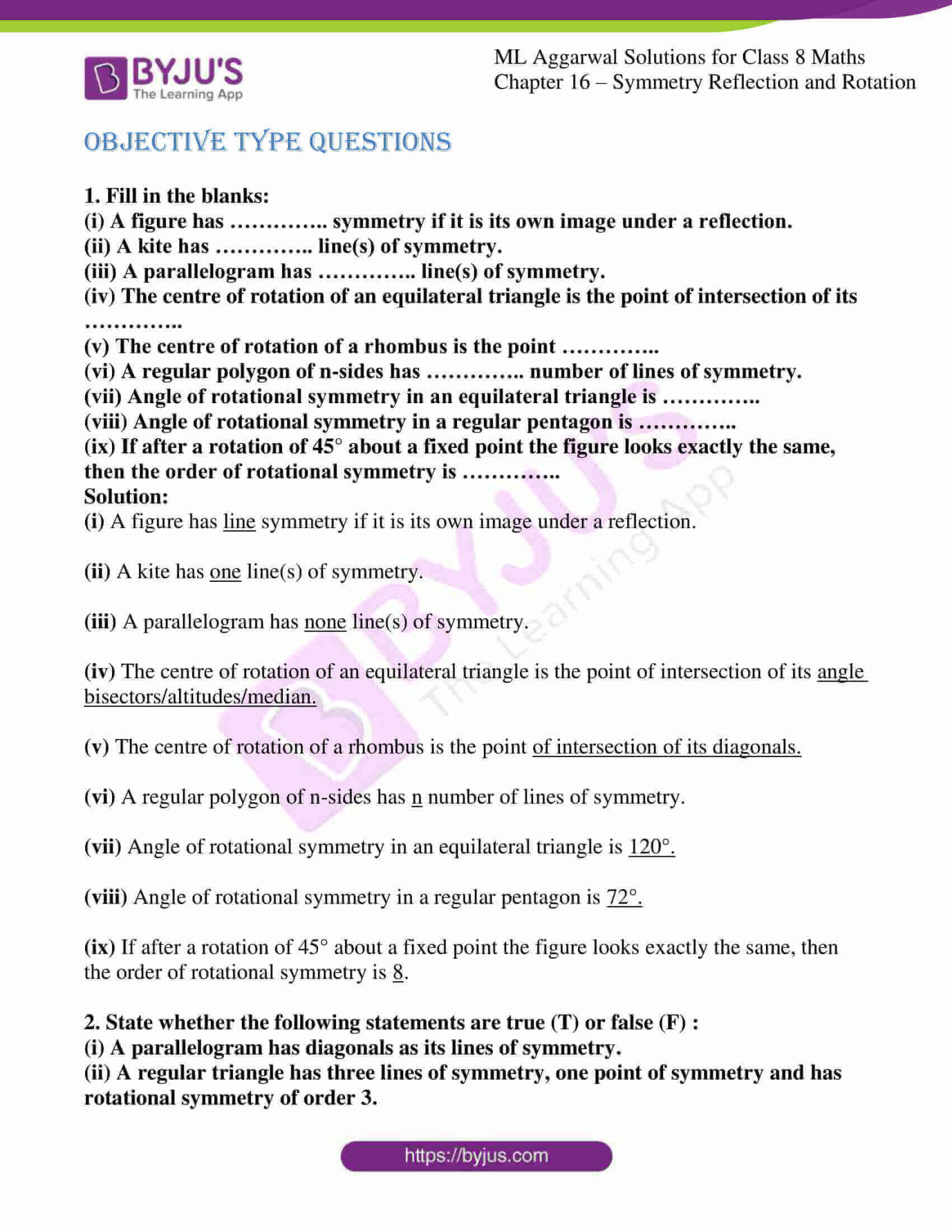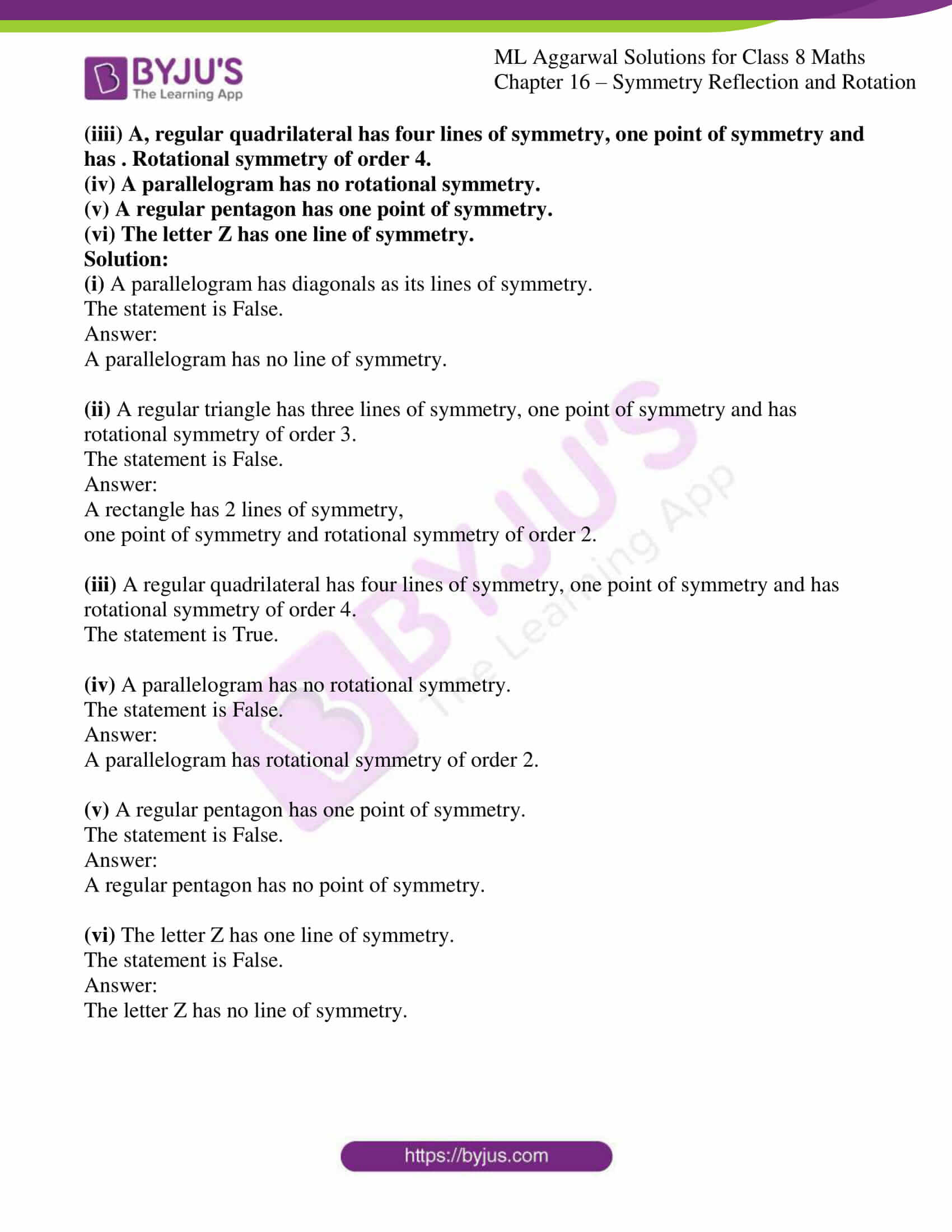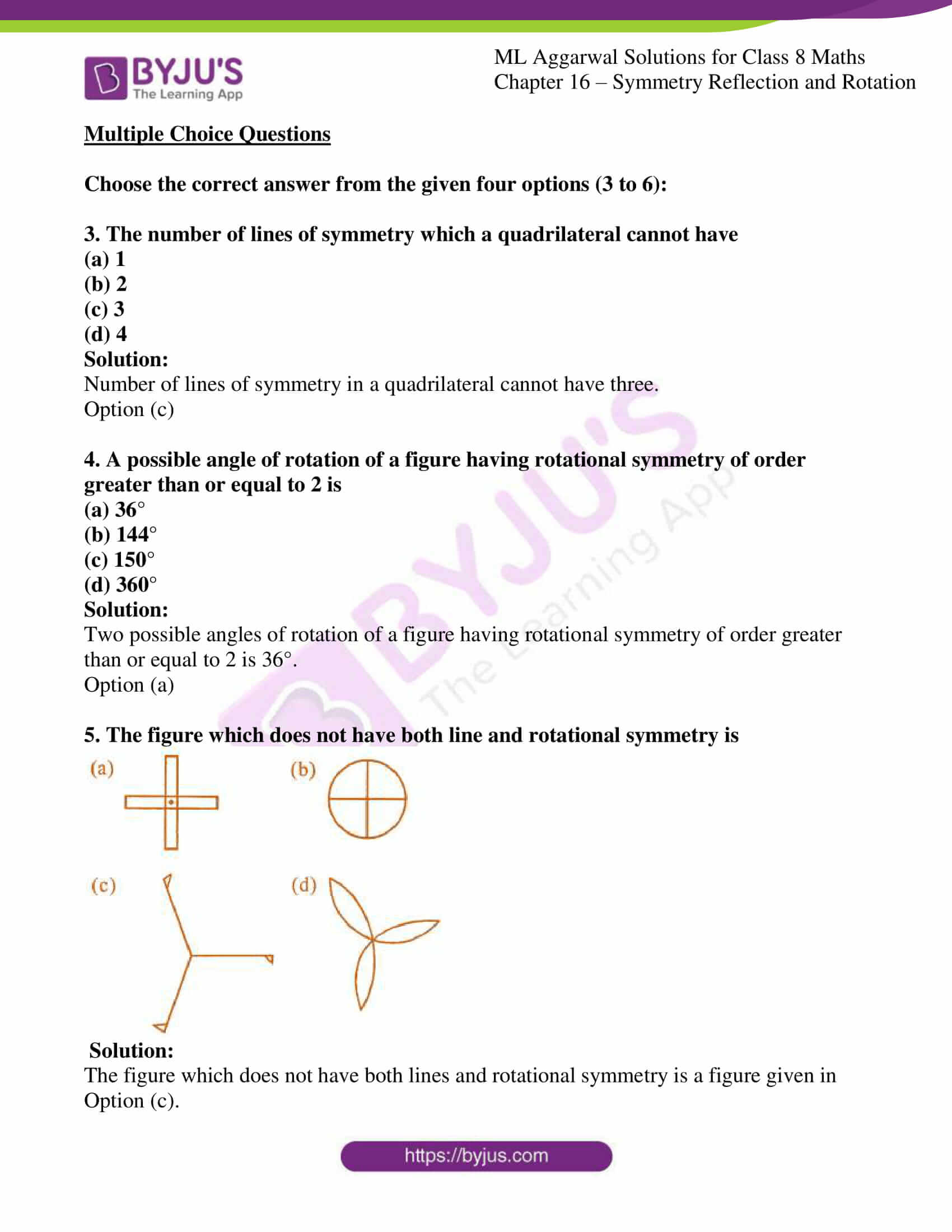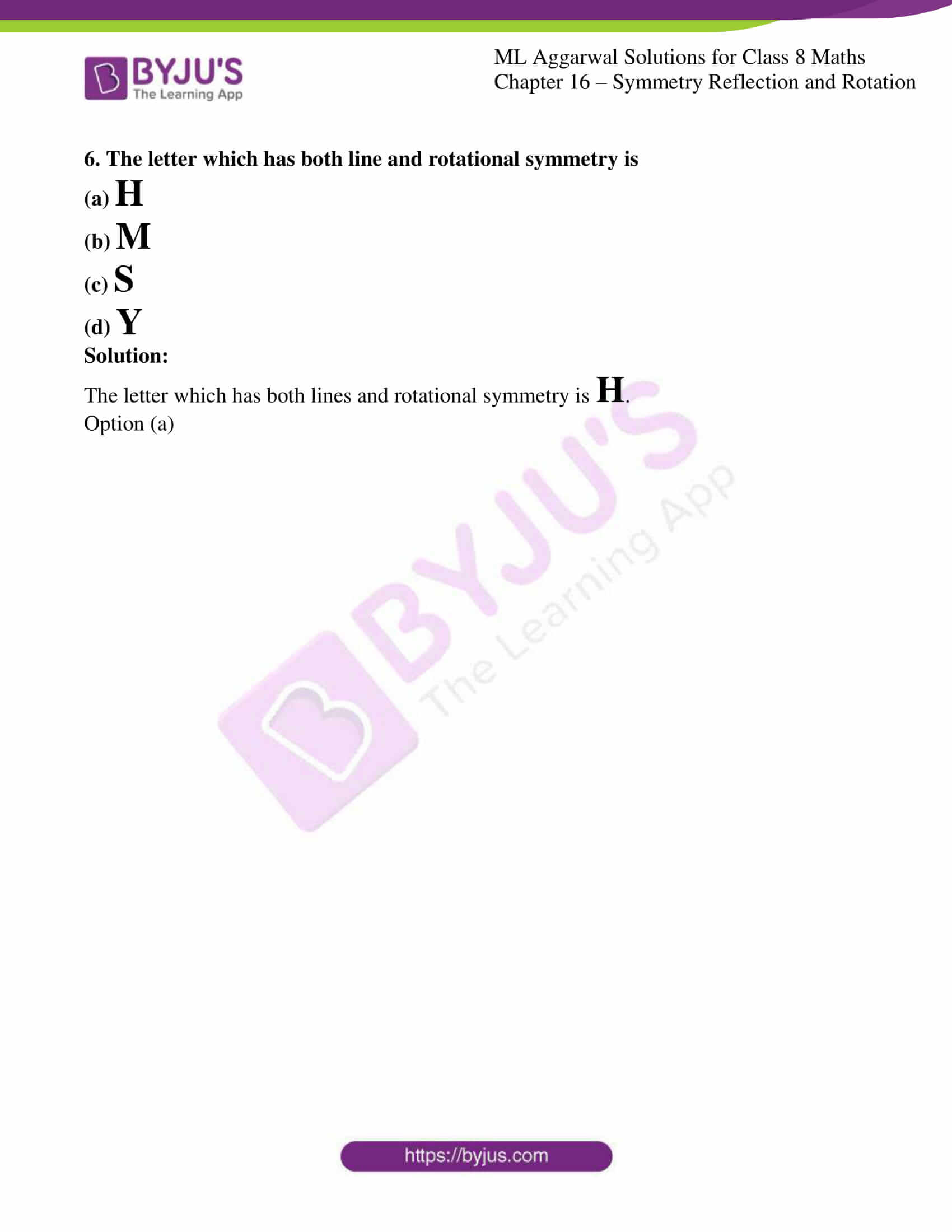### Access answers to ML Aggarwal Solutions for Class 8 Maths Chapter 16 – Symmetry Reflection and Rotation

EXERCISE 16

1. Draw the line or lines of symmetry, if any, of the following shapes and count their number: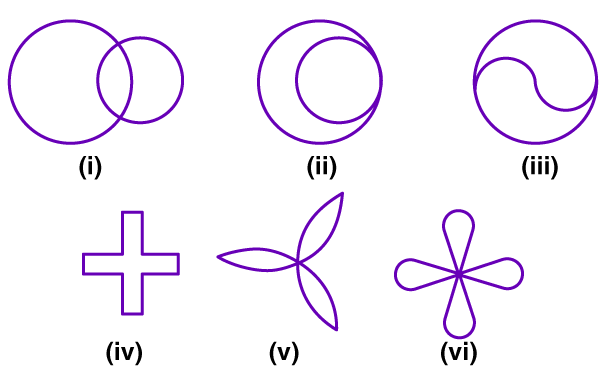Solution: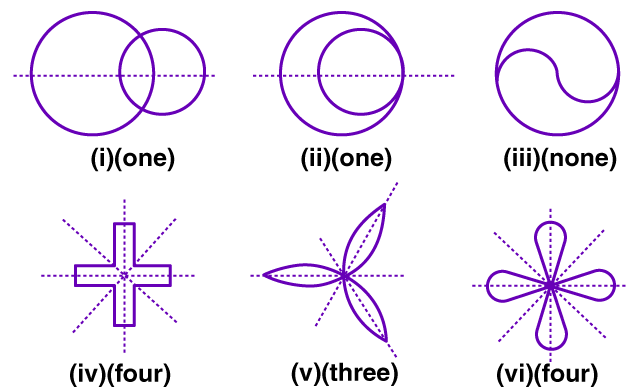2. For each of the given shape in question 1, find the order of the rotational symmetry (if any).
Solution:

(i) None

(ii) None

(iii) Two

(iv) Four

(v) Three

(vi) Four

3. Construct a rectangle ABCD such that AB = 4.5 cm and BC = 3 cm. Draw its line (or lines) of symmetry.

Solution:

Steps to construct:

Step 1: Draw a line segment AB = 4.5 cm.

Step 2: Draw a line BQ from point B making an angle of 90°.

Step 3: Taking B as centre and with radius = 3 cm, cut an arc on BQ, mark it as point C.

Step 4: Now taking point C as centre and radius = 4.5 cm, draw an arc and with A as centre and radius = 3cm, cutting the previous arc with marking that point as D.

Step 5: Join CD and AD.

Step 6: ABCD is a required rectangle.

The lines of symmetry: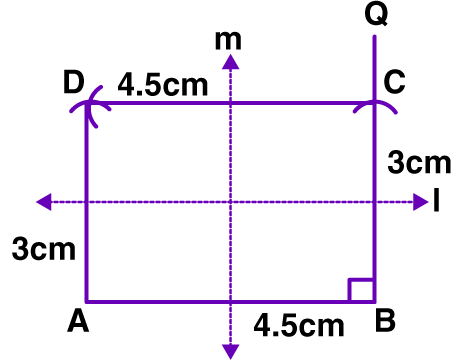The lines of symmetry = 2, i.e., the lines joining the midpoints of opposite sides.

4. Construct a rhombus ABCD with AB = 5.3 cm and ∠A = 60°. Draw its line (or lines) of symmetry.
Solution:

Steps to construct:

Step 1: Draw line segment AB = 5.3 cm.

Step 2: Construct an angle of 60° at point A.

Step 3: With A as centre, radius = 5.3 cm draw an arc on AX. Mark the point of intersection as D.

Step 4: With D as the centre and radius = 5.3 cm draw an arc. Mark that point as C.

Step 5: With B as centre and same radius, draw another arc, such that it intersects the previous at point C.

Step 6: Join CD and BC.

Step 7: ABCD is a required rhombus.

The lines of symmetry: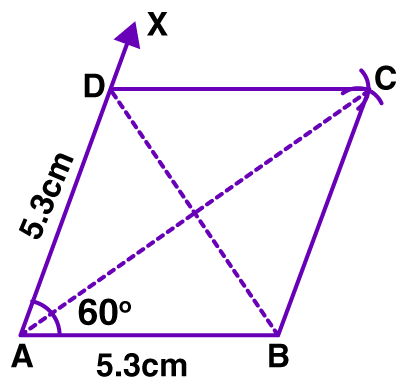Lines of symmetry in rhombus = 2, i.e., the diagonals of the rhombus.

Objective type Questions

1. Fill in the blanks:
(i) A figure has ………….. symmetry if it is its own image under a reflection.
(ii) A kite has ………….. line(s) of symmetry.
(iii) A parallelogram has ………….. line(s) of symmetry.
(iv) The centre of rotation of an equilateral triangle is the point of intersection of its …………..
(v) The centre of rotation of a rhombus is the point …………..
(vi) A regular polygon of n-sides has ………….. number of lines of symmetry.
(vii) Angle of rotational symmetry in an equilateral triangle is …………..
(viii) Angle of rotational symmetry in a regular pentagon is …………..
(ix) If after a rotation of 45° about a fixed point the figure looks exactly the same, then the order of rotational symmetry is …………..
Solution:

(i) A figure has line symmetry if it is its own image under a reflection.

(ii) A kite has one line(s) of symmetry.

(iii) A parallelogram has none line(s) of symmetry.

(iv) The centre of rotation of an equilateral triangle is the point of intersection of its angle bisectors/altitudes/median.

(v) The centre of rotation of a rhombus is the point of intersection of its diagonals.

(vi) A regular polygon of n-sides has n number of lines of symmetry.

(vii) Angle of rotational symmetry in an equilateral triangle is 120°.

(viii) Angle of rotational symmetry in a regular pentagon is 72°.

(ix) If after a rotation of 45° about a fixed point the figure looks exactly the same, then the order of rotational symmetry is 8.

2. State whether the following statements are true (T) or false (F) :
(i) A parallelogram has diagonals as its lines of symmetry.
(ii) A regular triangle has three lines of symmetry, one point of symmetry and has rotational symmetry of order 3.
(iiii) A, regular quadrilateral has four lines of symmetry, one point of symmetry and has . Rotational symmetry of order 4.
(iv) A parallelogram has no rotational symmetry.
(v) A regular pentagon has one point of symmetry.
(vi) The letter Z has one line of symmetry.
Solution:

(i) A parallelogram has diagonals as its lines of symmetry.

The statement is False.

A parallelogram has no line of symmetry.

(ii) A regular triangle has three lines of symmetry, one point of symmetry and has rotational symmetry of order 3.

The statement is False.

A rectangle has 2 lines of symmetry,

one point of symmetry and rotational symmetry of order 2.

(iii) A regular quadrilateral has four lines of symmetry, one point of symmetry and has rotational symmetry of order 4.

The statement is True.

(iv) A parallelogram has no rotational symmetry.

The statement is False.

A parallelogram has rotational symmetry of order 2.

(v) A regular pentagon has one point of symmetry.

The statement is False.

A regular pentagon has no point of symmetry.

(vi) The letter Z has one line of symmetry.

The statement is False.

The letter Z has no line of symmetry.

Multiple Choice Questions

Choose the correct answer from the given four options (3 to 6):

3. The number of lines of symmetry which a quadrilateral cannot have
(a) 1
(b) 2
(c) 3
(d) 4
Solution:

Number of lines of symmetry in a quadrilateral cannot have three.

Option (c)

4. A possible angle of rotation of a figure having rotational symmetry of order greater than or equal to 2 is
(a) 36°
(b) 144°
(c) 150°
(d) 360°
Solution:

Two possible angles of rotation of a figure having rotational symmetry of order greater than or equal to 2 is 36°.

Option (a)

5. The figure which does not have both line and rotational symmetry is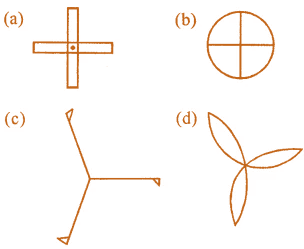Solution:

The figure which does not have both lines and rotational symmetry is a figure given in

Option (c).

6. The letter which has both line and rotational symmetry is

(a) H

(b) M

(c) S

(d) Y

Solution:

The letter which has both lines and rotational symmetry is H.

Option (a)